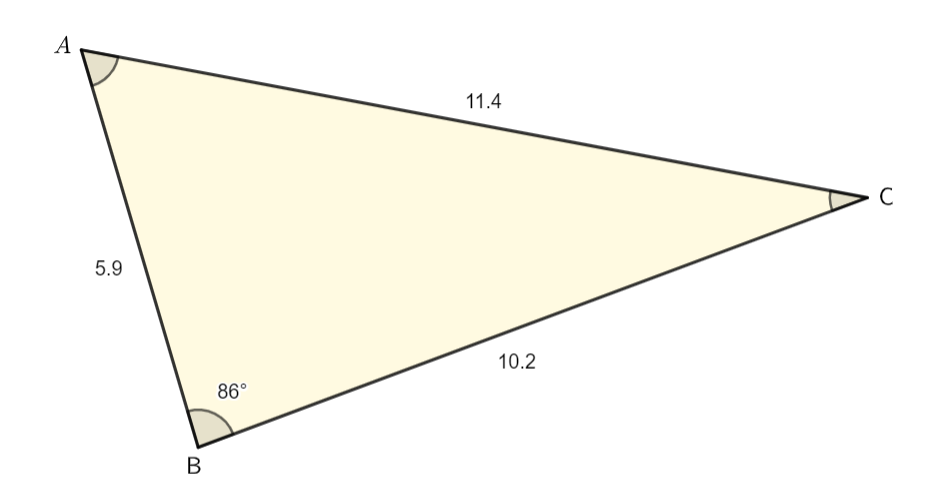Law of Sines homework Help at TutorEye

## Top Questions

t schedule 3 people for the day my % scheduled is 78.57% My question is how did the computer come up with the 78.57%?
View More

I would love to get a written solution. I did the other proof tree that you can see in the attaached files. So its supposed to look like that Thank You.
View More

xtbook. i really need in in half an hour
View More

## Law of Sines:

It states a relation between sides and the angle opposite to that side of a triangle.

The ratio of sides of the triangle and sine of the corresponding angle to that side, will remain the same for all three sides of the triangle.

a/sinA = b/sinB = c/sinC = 2R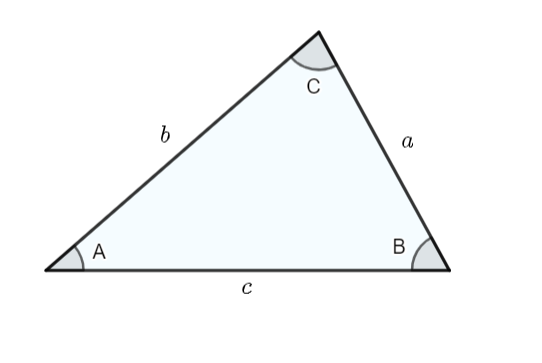## Law of Sines Sample Questions:

Question 1: Find b using the following triangle. Write your answer to the nearest whole number.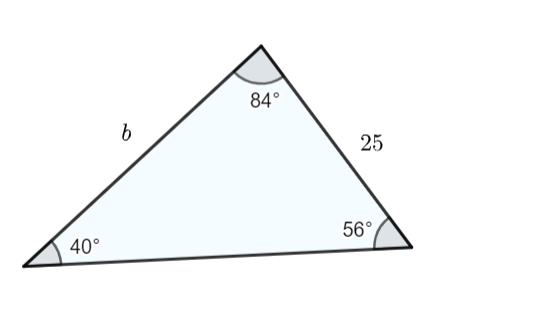Explanation: We will use the Law of Sine here.

Question 2: Find a using the following triangle. Write your answer to the nearest whole number.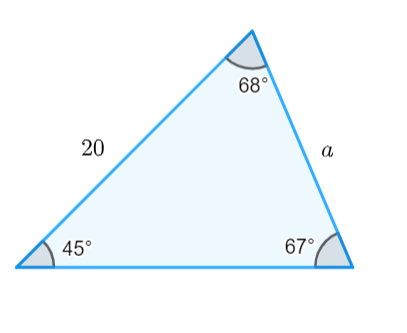Explanation: We will use the Law of Sine here.

Question 3: Find c using the following triangle. Write your answer to the nearest whole number.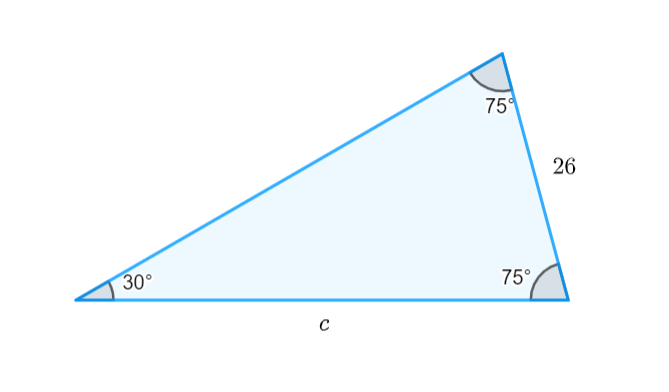Explanation: We will use the Law of Sine here.

Question 4: Find the measure of m∠B using the following triangle. Write your answer to the nearest degree.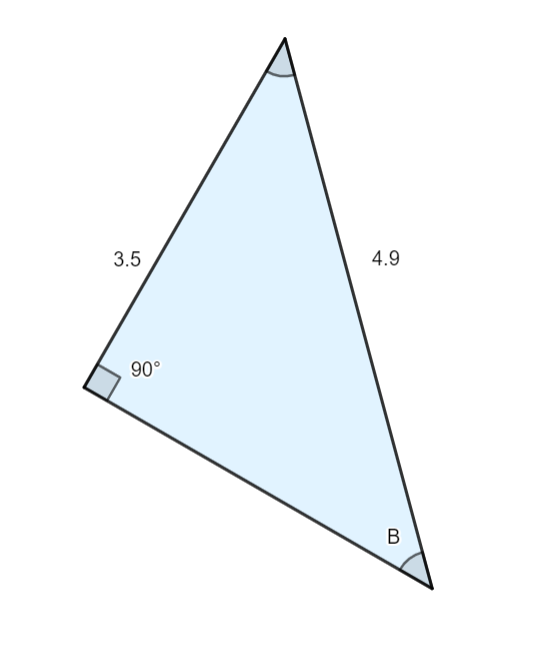Explanation: We will use the Law of Sine here.

Question 5: Find the measure of m∠A using the following triangle. Write your answer to the nearest degree.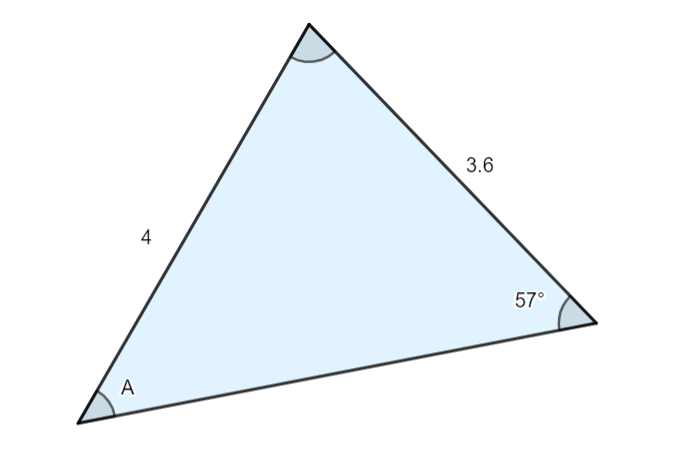Explanation: We will use the Law of Sine here.

Question 6: Find the measure of mC using the following details.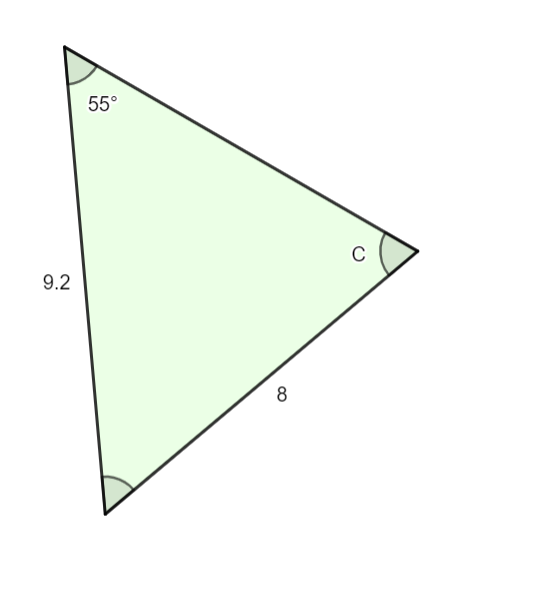Explanation: We will use the Law of Sine here.

Question 7: For the following ABC, find mB. Round to the nearest degree.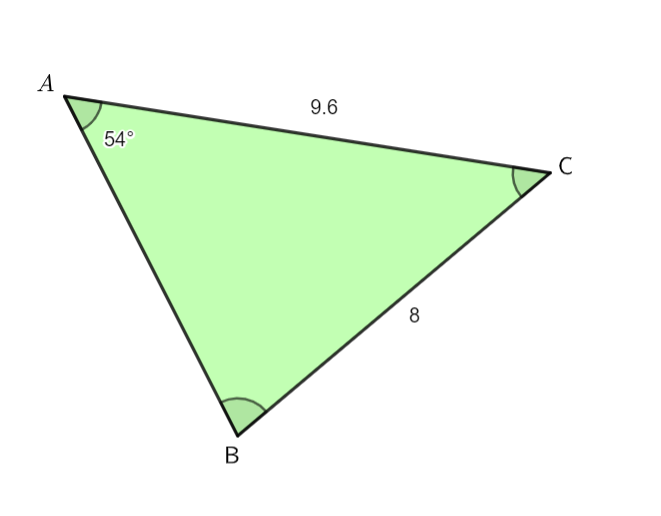Explanation: We will use the Law of Sine here.

Question 8: Find a using the following ABC. Write your answer to the nearest tenth.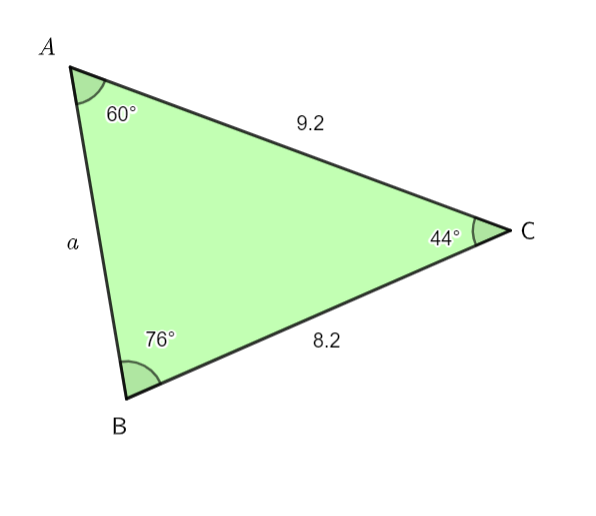Explanation: We will use the Law of Sine here.

Question 9: Find b using the following ABC. Write your answer to the nearest tenth.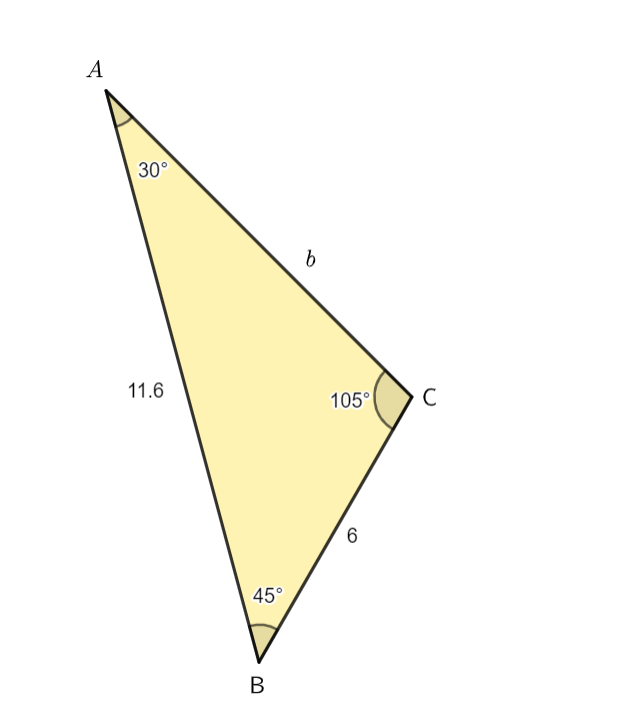Explanation: We will use the Law of Sine here.

Question 10: Find the measure of m∠C using the following ABC.